# Class 12 Maths NCERT Solutions for Chapter 7 Integrals Exercise 7.11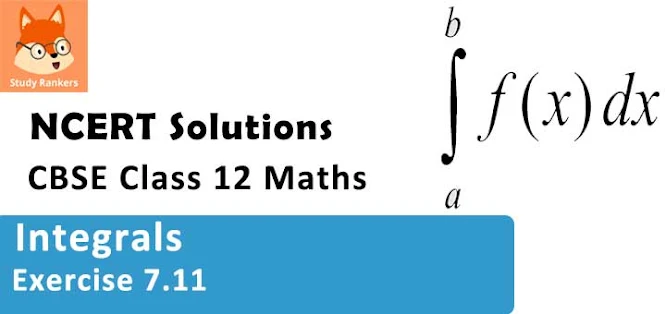### Integrals Exercise 7.11 Solutions

1. By using the properties of definite integrals, evaluate the integrals ∫0π/2 cos2x dx

Solution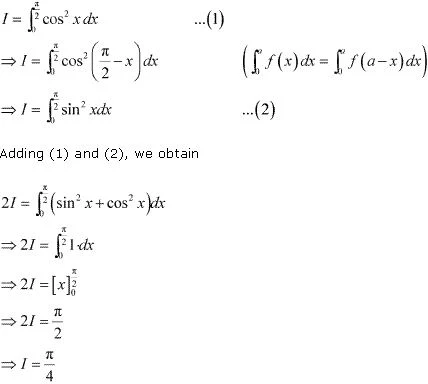2. By using the properties of definite integrals, evaluate the integrals  ∫0π/2 √(sin x)/(√sinx + √cos x)dx
Solution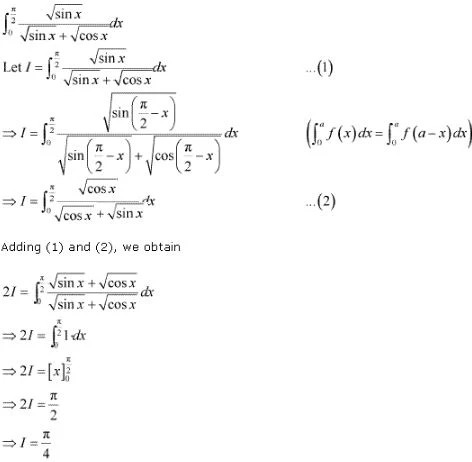3. By using the properties of definite integrals, evaluate the integrals  ∫0π/2 (sin3/2 x dx)/(sin3/2 x + cos3/2 x)
Solution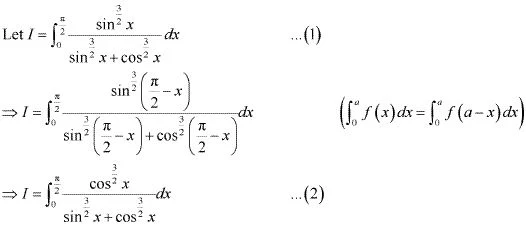Adding (1) and (2), we obtain4. By using the properties of definite integrals, evaluate the integrals
0π/2 (cos5 x dx)/(sin5x + cos5x)
Solution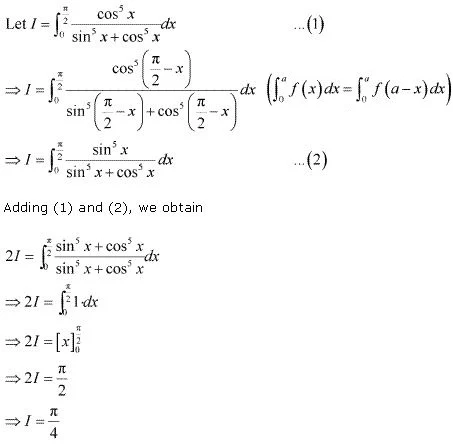5. By using the properties of definite integrals, evaluate the integrals
-55 |x + 2| dx
Solution
Let I =  ∫-55 |x + 2| dx
It can be seen that (x + 2) ≤ 0 on [-5, -2] and (x + 2) ≥ 0 on [-2, 5].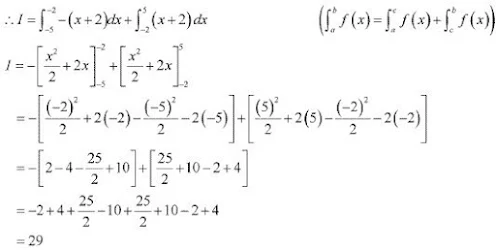6. By using the properties of definite integrals, evaluate the integrals
28 |x - 5| dx
Solution
Let I = ∫28 |x - 5| dx
It can be seen that (x - 5) ≤ 0 on [2, 5] and (x - 5) ≥ 0 on [5, 8].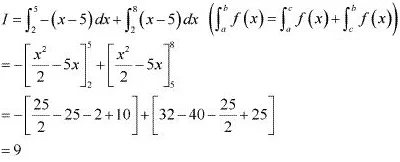7. By using the properties of definite integrals, evaluate the integrals ∫04 x(1 - x)n  dx
Solution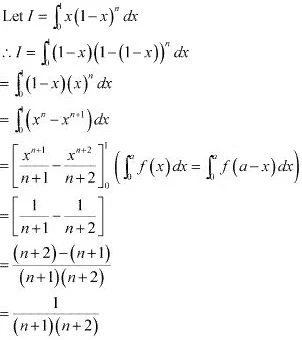8. By using the properties of definite integrals, evaluate the integrals ∫0π/4 log(1 + tan x) dx
Solution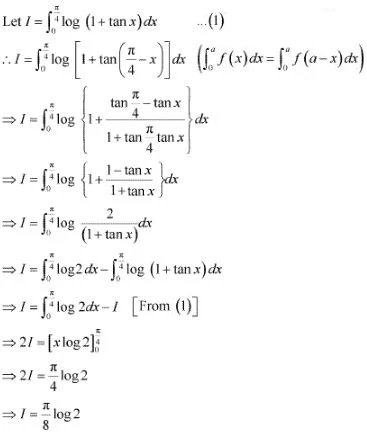9. By using the properties of definite integrals, evaluate the integrals ∫02 x√(2 - x) dx
Solution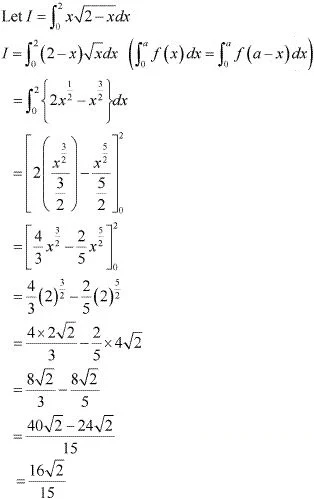10. By using the properties of definite integrals, evaluate the integrals ∫0π/2 (2 log sin x - log sin 2x) dx
Solution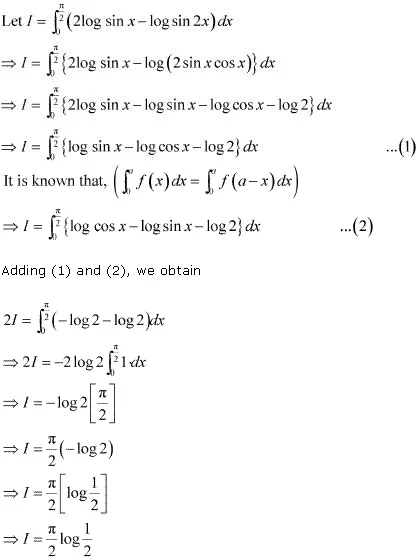11. By using the properties of definite integrals, evaluate the integrals ∫-π/2π/2 sin2 x dx
Solution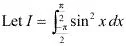As sin2 (-x) = [sin (-x)]2 = (-sin x)2  = sin2 x , therefore, sin2 x is an even function.
It is known that if f(x) is an even function, then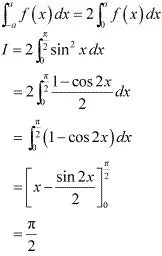12. By using the properties of definite integrals, evaluate the integrals ∫0π xdx/(1 + sin x)
Solution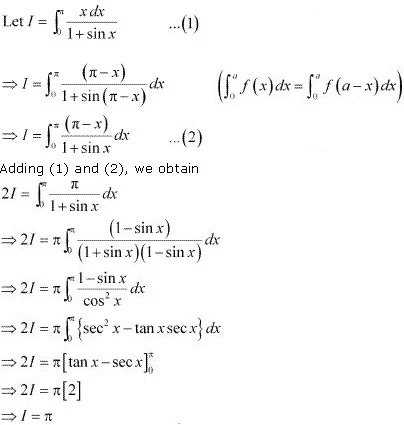13. By using the properties of definite integrals, evaluate the integrals ∫π/2π/2 sin7x dx
Solution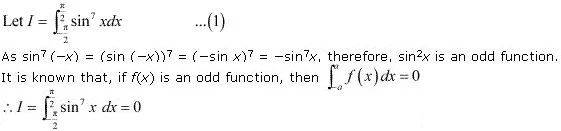14. By using the properties of definite integrals, evaluate the integrals, ∫02x cos5 x dx
Solution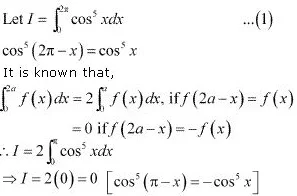15. By using the properties of definite integrals, evaluate the integrals
0π [(sin x - cos x)/(1 + sin x cos x)] dx
Solution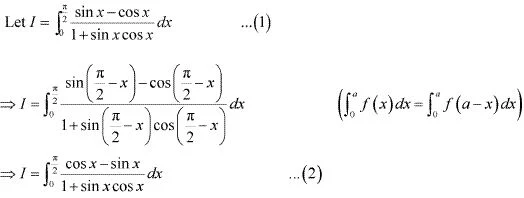Adding (1) and (2), we obtain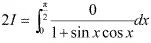⇒ l = 0

16. By using the properties of definite integrals, evaluate the integrals ∫0π  log(1 + cos x) dx
Solution
Let I = ∫0π  log(1 + cos x) dx ...(1)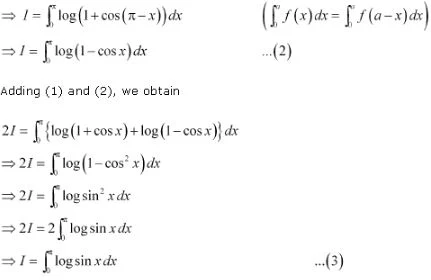sin(π - x) = sin x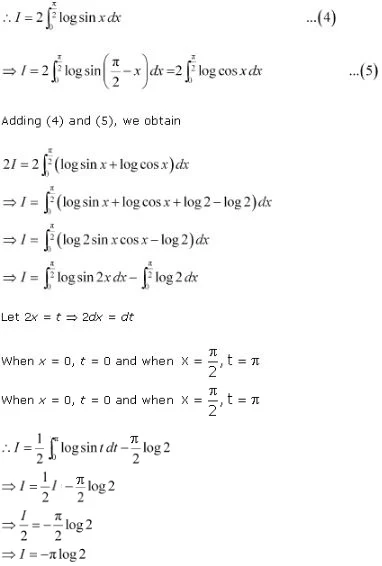17. By using the properties of definite integrals, evaluate the integrals
0a √x/[√x + (√a - x)] dx
Solution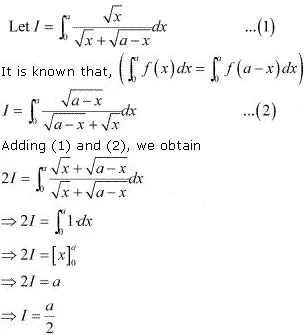18. By using the properties of definite integrals, evaluate the integrals
04 |x - 1| dx
Solution
I =  ∫04 |x - 1| dx
It can be seen that, (x - 1) ≤ 0 when 0 ≤ x ≤ 1 and (x - 1) ≥ 0 when 1 ≤ x ≤ 4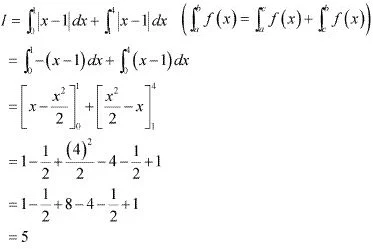19. Show that ∫0a f(x) g(x) dx = 2 ∫0a f(x)  dx if f and g are defined as f(x) = f(a - x) and g(x) + g(a - x) = 4
Solution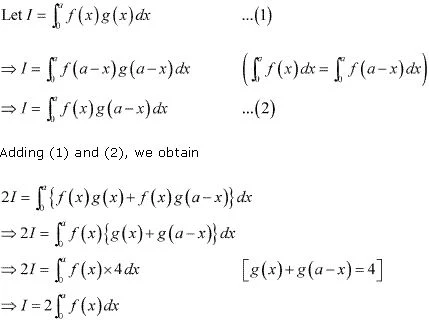20. Choose the correct The value of  ∫-π/2π/2  (x3 + x cos x + tan5 x + 1) dx is
(A) 0
(B) 2
(C) π
(D) 1
Solution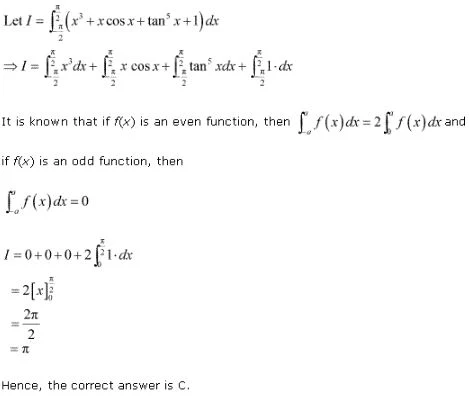21. Choose the correct The value of ∫0π/2 log  [(4 + 3 sinx)/(4 + 3 cos)] dx is
(A) 2
(B) 3/4
(C) 0
(D) -2
Solution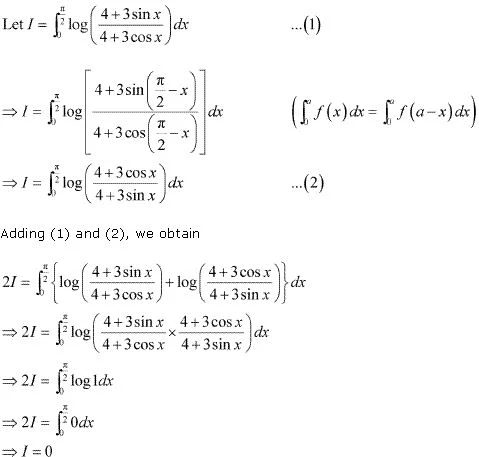Hence, the correct answer is C.